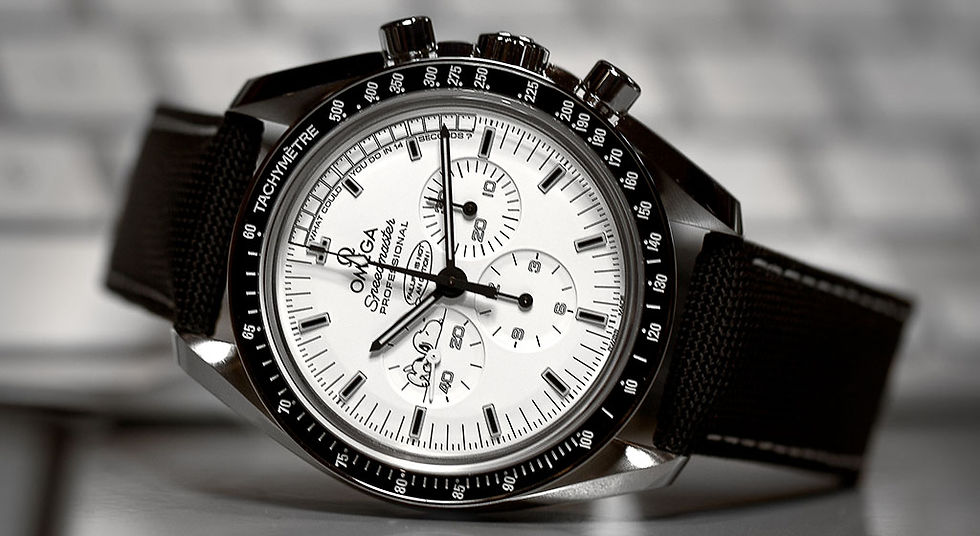top of pageSearch
• GWSC Team

# How To Use A Tachymeter

In our previous article 'A Guide To Chronographs', we introduced the tachymeter – measurements etched around the bezel of a watch or the outermost edge of the watch's main dial. Using the measurements, one will be able to obtain more complex measurements such as speed and distance.

For this article, we will focus on how to use a fixed tachymeter to determine speed and distance.Credits to: Gov Berg Watches

The term ‘tachymeter’ actually originated from the Greek words ‘tachos’ (speed) and ‘metron’ (measure). The base units for the tachymeter scale use either miles or kilometres. Take note that a tachymeter can only measure speeds for distances up to 1 full mile or kilometre. It will require some simple maths to measure speed for objects that travel shorter or longer distances.

The values on the tachymeter scale are derived by dividing 3,600 (number of seconds in an hour) by the elapsed time in seconds. The scale allows you to convert elapsed time (in seconds per unit) to speed (in units per hour).

First, you need to time the duration taken for an object to cover a 1 mile/km. Then based on what’s indicated by the watch’s chronograph hand, read off the tachymeter scale to obtain the speed.

Most tachymeter scales only work for elapsed time between 7.2 seconds to 60 seconds. As seen in the picture below, the tachymeter scale (on the bezel) begins at 500 around the 7.2 second mark. For an elapsed time of less than 7.2 seconds, it will not be possible to determine speed. For an elapsed time of more than 60 seconds, you can still use the tachymeter to determine speed, but will require some additional maths.Credits to: Art of Manliness

Firstly, we will show how speed is calculated.

1. Less than 60 seconds taken for a 1km distance

For example, you are trying to calculate the speed of a race car travelling from point A to point B, over a distance of 1km. When the race car starts at point A, you will press start on your chronograph and then stop the chronograph when the race car reaches point B. Let’s say the race car took 20 seconds to cover this distance. Using the tachymeter scale, at time = 20 seconds, it reads 180.

This means that the average speed of the race car is 180 km/h.

2. Less than 60 seconds taken, but less/more than 1km

Now let’s have the next example where a runner takes 20 seconds to run 200m. Reading off the tachymeter scale, you have 180 km/h. But that’s incorrect as the race was only 200m, which is one-fifth of 1km (1000m). Hence to find the average speed of the runner, you will have to find out what is one-fifth of 180 km/h.

This will give you an average speed of 36 km/h.

3. More than 60 seconds taken

For this last example, let’s say it takes 100 seconds for a fast food restaurant to make 1 burger. Since 100 seconds exceed the tachymeter’s limit of 60 seconds, we will not be able to read off the tachymeter scale straightaway. Instead, let’s take it as the restaurant needs 50 seconds to make ½ a burger. 50 seconds is within the range of the tachymeter scale. At 50 seconds, the scale reads 72.

This means an average of 72 half burgers, or 36 whole burgers are being made every hour.

Next, we can also use the tachymeter to calculate distance – a useful tool when you know your speed but have no distance indicators.

For instance, you know the speed is 80 miles/hr. When the seconds hand point to 80 on the tachymeter scale, it indicates that you have ran one mile.

As the tachymeter scale ends at 60, this tool works only if you are travelling at speeds over 60, regardless of the unit of measurement. If you are travelling at a lower speed, for example, 50 miles per hour, you will have to multiply the speed so that it reaches the valid range.

Multiplying 50 miles/hour by 2 will give you 100 miles/hr. When the tachymeter scale reaches 100 on the scale, divide the distance of 1 mile by the number multiplied (2). This means that you have covered ½ a mile.

With some simple maths manipulations, you can use the tachymeter to work out speeds and distances covered by objects.

That being said, we know that understanding how a tachymeter works might be a tad confusing at the start. But with practise and a good understanding of how time, speed and distance relate to one another, in no time you will be able to impress your friends with your tachymeter knowledge.